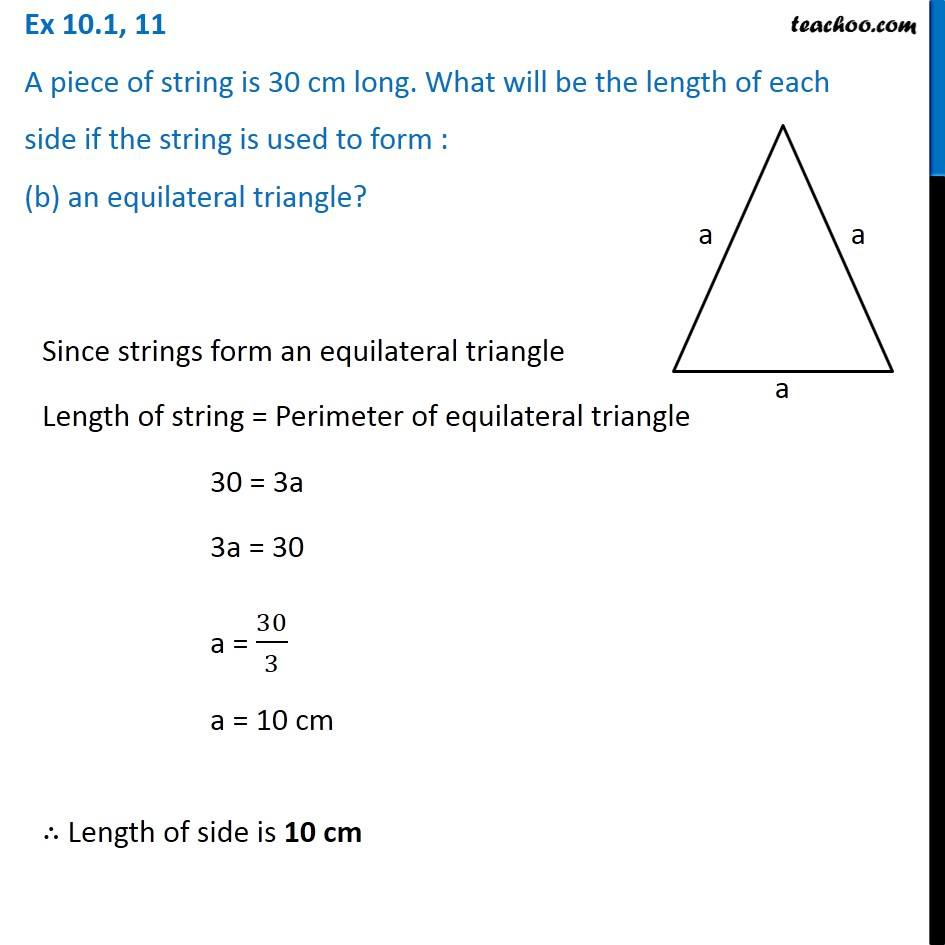Ex 10.1

Chapter 10 Class 6 Mensuration
Serial order wiseLearn in your speed, with individual attention - Teachoo Maths 1-on-1 Class

### Transcript

Ex 10.1, 11 A piece of string is 30 cm long. What will be the length of each side if the string is used to form : (b) an equilateral triangle? Since strings form an equilateral triangle Length of string = Perimeter of equilateral triangle 30 = 3a 3a = 30 a = 30/3 a = 10 cm ∴ Length of side is 10 cm